# Analysis Techniques: Flood Frequency Analysis

View and print this webpage as a pdf file.

What is it?

Flood frequency analyses are used to predict design floods for sites along a river. The technique involves using observed annual peak flow discharge data to calculate statistical information such as mean values, standard deviations, skewness, and recurrence intervals. These statistical data are then used to construct frequency distributions, which are graphs and tables that tell the likelihood of various discharges as a function of recurrence interval or exceedence probability.

Flood frequency distributions can take on many forms according to the equations used to carry out the statistical analyses. Four of the common forms are:

Each distribution can be used to predict design floods; however, there are advantages and disadvantages of each technique. Click on the above links to learn more about each technique. According to the U.S. Water Advisory Committee on Water Data (1982), the Log-Pearson Type III Distribution is the recommended technique for flood frequency analysis. Therefore, this analysis is examined in detail here with a step-by-step tutorial.

### Log-Pearson Type III Distribution

What is it?

The Log-Pearson Type III distribution is a statistical technique for fitting frequency distribution data to predict the design flood for a river at some site. Once the statistical information is calculated for the river site, a frequency distribution can be constructed.  The probabilities of floods of various sizes can be extracted from the curve. The advantage of this particular technique is that extrapolation can be made of the values for events with return periods well beyond the observed flood events. This technique is the standard technique used by Federal Agencies in the United States.

How is it calculated?

The Log-Pearson Type III distribution is calculated using the general equation: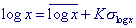where x is the flood discharge value of some specified probability,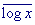is the average of the log x discharge values, K is a frequency factor, and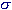is the standard deviation of the log x values. The frequency factor K is a function of the skewness coefficient and return period and can be found using the frequency factor table. The flood magnitudes for the various return periods are found by solving the general equation.  The mean, variance, and standard deviation of the data can be calculated using the two formulas below.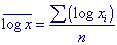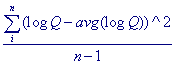and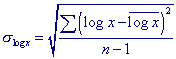or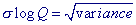Next, the skewness coefficient  Cs  can be calculated as follows: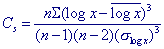where n is the number of entries, x  the flood of some specified probability and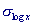is the standard deviation.  Excel functions can also be used to calculate the variance (=VAR( ) ), standard deviation (=STDEV( ) ), and skewness coefficient (=SKEW( ) ).

The skewness estimate (Cs) computed using the equation above is called the station estimate, meaning that the estimate incorporates data values only from the gaging station of interest.

Error and bias in the skewness estimate increase as the number of observations (n) decreases.  The Bulletin 17B method recommended by the Interagency Advisory Committee on Water Data (IACWD) uses a generalized estimate of the coefficient of skewness, Cw (for instantaneous peak flow data only), based on the equation:

Cw = WCs + (1-W)Cm

where W is a weighting factor, Cs is the coefficient of skewness computed using the sample data, and Cm is a regional skewness, which is determined from a map.

The weighting factor W is calculated to minimize the variance of Cw, where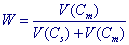Determination of W requires knowledge of variance of Cm [V(Cm)] and variance of Cs[V(Cs)].  V(Cm) has been estimated from the map of skew coefficients for the United States as 0.302 (IACWD, 1982). This simplifies the denominator of the above equation by substitution of 0.302 for V(Cm).

The variance of the station skew Cs for log Pearson type 3 random variables can be obtained from the results of Monte Carlo experiments by Wallis et al. (1974).  They showed that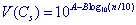where

A = -0.33 + 0.08 | Cs| if | Cs |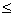0.90 or
A = -0.52 + 0.30 | C s | if | C s | > 0.90,
B = 0.94 - 0.26 | C s | if | C s |1.50 or
B = 0.55 if | C s | > 1.50

in which | C s | is the absolute value of the station skew (used as an estimate of population skew) and n is the record length in years.

The coefficient K is then found using tabulated values according to Cw and the return period for each discharge.

For a more detailed description of this method, please refer to the following text:

Bedient, Philip B. and Wayne C. Huber. Hydrology and Floodplain Analysis. Prentice-Hall, Inc., Upper Saddle River, 2002.